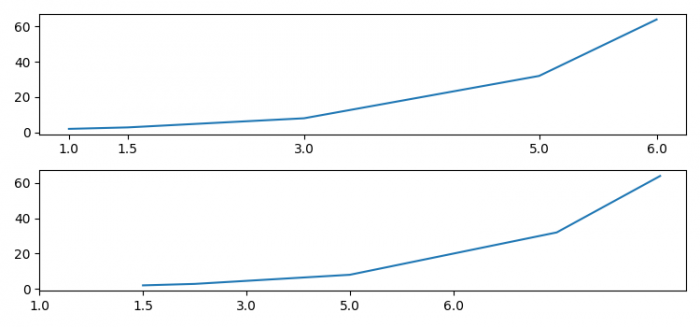# How to make xticks evenly spaced despite their values? (Matplotlib)

To make xticks evenly spaced despite their values, we can use set_ticks() and set_ticklabels() methods.

## Steps

• Set the figure size and adjust the padding between and around the subplots.
• Create x and y data points using numpy.
• Create a figure and a set of subplots using subplots() method.
• Plot x and y data points on axis 1.
• Set xticks using xaxis.set_ticks() method.
• Plot x and y data points on axis 2.
• Set xticks and ticklabels using xaxis.set_ticks() and xaxis.set_ticklabels() method.
• To display the figure, use show() method.

## Example

import numpy as np
from matplotlib import pyplot as plt

plt.rcParams["figure.figsize"] = [7.50, 3.50]
plt.rcParams["figure.autolayout"] = True

x = np.array([1, 1.5, 3, 5, 6])
y = np.power(2, x)

fig, (ax1, ax2) = plt.subplots(2, 1)
ax1.plot(x, y)
ax1.xaxis.set_ticks(x)

ax2.plot(x, y)
ax2.xaxis.set_ticks(range(len(x)))
ax2.xaxis.set_ticklabels(x)

plt.show()

## Output